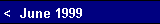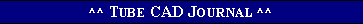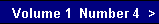CMRR: common mode rejection   What if the same signal is presented to both inputs at once? Just what we want to happen: nothing (for the most part that is). Common mode signals should be ignored by the output stage. Just as a tug of war between equally strong opponents results in a draw, the noise presented equally to the grids of the output stage should cancel because of the equal increases and decreases in current flow through the devices. Of course, part tolerance and triode mu mismatch will compromise the cancellation. Still, with just two triodes we have been able to steal the one big advantage of an output transformer: common mode rejection. Output Impedance    What is the output impedance of this circuit, considering  the two resistor networks wrapped about the circuit? Unity is the answer. By this we mean that the two triodes yield an output impedance equal to one triode configured as a Cathode Follower. To understand how this result may occur, imagine a positive voltage pulse being fed into the output of our circuit. This pulse would provoke a change in current flow through the circuit that would work to eliminate the pulse. Since the tubes only see half of the pulse at their grids, only half of the potential change in current occurs per tube. Two times one half equals unity. Thus the voltage divided by the change in current equals the output impedance.    Once again, but in greater detail. We assume that the inputs are effectively grounded by the preceding stage's low output impedance. We also assume the cathode resistors are
 bypassed to make the circuit analyses simpler. Lets say a 1 volt pulse is forced into the output by a very buffed, low output impedance solid-state amplifier. The cathode-plate connection is now 1 volt higher. This 1 volt increase is relayed through the top resistor network to the top tubes grid. Since the two resistors are wired in series, not all the voltage can present itself to the grid; in fact, as the two resistors are equal in value, only half of the 1 volt increase reaches the top grid. This is equivalent to the grid having been driven ½ volt negative relative to the cathode, as the cathode has moved up 1 volt and the grid has only moved up ½ volt. The top triode will conduct less as a result of the negative voltage on its grid. How much? The transconductance of the triode times ½ volt is basically the amount of decreased current. In the case of a 6922 with a Gm of 10 mA per volt, the current will decrease by 5 mA.   On the bottom tube, the positive pulse is also relayed to its grid via a resistor network. Once again only half of the pulse makes it to the grid. But this time the current increases by the Gm times the ½ volt positive pulse. In the case of a 6922, the current will increase by 5 mA.   The sum of the positive increase in current flow by the bottom tube and the negative decrease in current flow by the top tube is what the solid-state power amplifier must be able to source to maintain the pulse in the face of the change in current flowing through the tubes. Assume the at idle our balanced converter draws 10 mA. But in the presence of the 1 volt pulse, the top tube draws 5 mA less, which leaves it with 5 mA of current flow; the bottom tube's current draw increases by 5 mA, which leaves it with 15 mA of currentwww.tubecad.com   Copyright © 1999 GlassWare   All Rights Reserved
 pg. 13